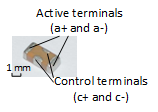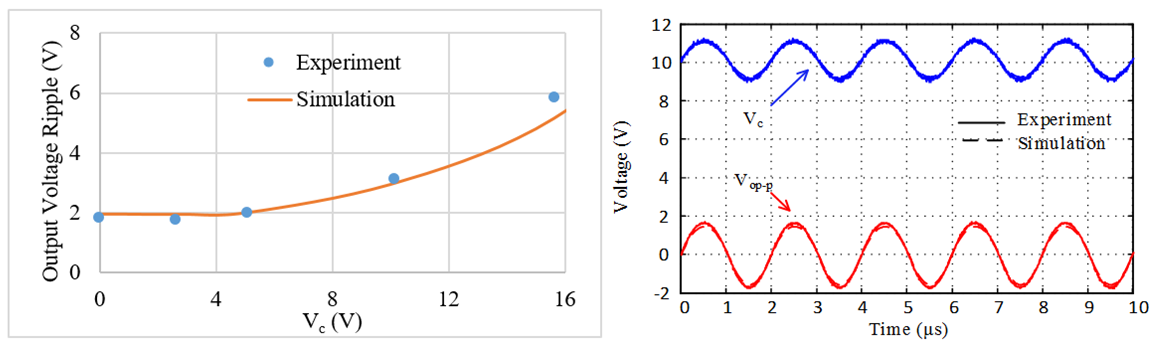LIBRARY

# Modeling of Voltage-Controlled Capacitor (VCC) in Dc-Dc Converters

Year: 2017 | Author: Lujie Zhang | Paper: D2.2Fig. 1. Prototype of the VCC
Capacitors transfer power, store energy, and filter harmonics in power electronics applications. Steady-state performance (e.g., efficiency and voltage ripple) and dynamic performance (e.g., overshoot and settling time) of dc-dc converters are determined by capacitor properties such as capacitance, ESR, voltage coefficient, and temperature coefficient. Capacitance usually drifts with the bias voltage because the permittivity of dielectric materials in a capacitor depends on the value of the applied electric field. Either a capacitor with stable permittivity (e.g., NP0) should be used, or the voltage across that capacitor should be well controlled to compensate for such undesired capacitance change, which both limits component selection and increases design complexity.

This paper presents a four-terminal VCC that varies from 20% to 100% of the rated capacitance (1 μF) with a control voltage from half of the voltage rating to 0 V. The prototype is shown in Fig. 1.

Models of nonlinear capacitors vary from the application. The C-V model is commonly used in dc-dc converters where the ac component is quite small compared with the dc component in capacitor voltages. In the C-V model, a capacitor current is generated only by a voltage change, such as a fixed capacitor even though a capacitance change is not considered another current source. Only the C-V curve, which can be measured by an impedance analyzer, is required in this method.

The VC was employed as an output filter of a buck converter with input of 12 V, output of 5 V, and switching frequency of 500 kHz to demonstrate its impact of controllability on the voltage ripple. The experiment hardware is shown in Fig.2. Simulation based on the C-V model showed an 8% error with an experimental waveform, which verified the effectiveness of the model in dc-dc converters. The comparison of simulation and experiment results are shown in Fig. 3. Future work will be focused on the design of the driver and closed-loop control of the VCC in other applications and circuit topologies.Fig. 2. Experiment hardware of buck converter with VCC in Fig. 1 as output capacitor.Fig. 3. Comparison of simulation and experiment results in Fig. 2 with (a) output voltage ripple with VC changing from 0 V to 12 V and (b) time-domain waveform with VC = 10 V.